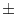Next: Algorithms for Planar Graphs Up: Graph Algorithms Previous: Minimum Spanning Trees (   Contents   Index

# Euler Tours ( euler_tour )

An Euler tour in an undirected graph G is a cycle using every edge of G exactly once. A graph has an Euler tour if it is connected and the degree of every vertex is even.

 bool Euler_Tour(const graph& G, list >& T) The function returns true if the underlying undirected version of graph G has an Euler tour. The Euler tour is returned in T. The items in T are of the form (e,+ 1), where the second component indicates the traversal direction d of the edge. If d = + 1, the edge is traversed in forward direction, and if d = - 1, the edge is traversed in reverse direction. The running time is O(n + m). bool Check_Euler_Tour(const graph& G, const list >& T) returns true if T is an Euler tour in G. The running time is O(n + m). bool Euler_Tour(graph& G, list& T) The function returns true if the underlying undirected version of G has an Euler tour. G is reoriented such that every node has indegree equal to its outdegree and an Euler tour (of the reoriented graph) is returned in T. The running time is O(n + m). bool Check_Euler_Tour(const graph& G, const list& T) returns true if T is an Euler tour in the directed graph G. The running time is O(n + m).Next: Algorithms for Planar Graphs Up: Graph Algorithms Previous: Minimum Spanning Trees (   Contents   Index